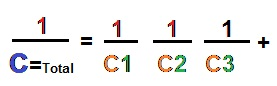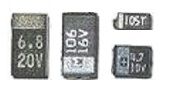# How to test Electrolytic Capacitor

What is capacitor:-Capacitor is an electronic component that stores electric charge. The capacitor is made of 2 close conductors (usually plates) that are separated by a dielectric material. The plates accumulate electric charge when connected to power source. One plate accumulates positive charge and the other plate accumulates negative charge.
Capacitor Symbols.Capacitors are the devices which can store electric charge   They are used in
1.Tuned circuits,
2.Timing circuits,
3.Filters,
4.Amplifier circuits,
5.Oscillator circuits,
Capacitors are generally classified according to the dielectric use. Most commonly used dielectrics are,• Air,
• Electrolyte,
• Ceramic,
• Plastic, Mica and paper.

IMPORTANT:- only electrolytic capacitors have polarity.
Fixed capacitors: are
1. Aluminium type
2. Tantalum type
a. Ceramic capacitor (Disc capacitor)
b. Tubular capacitor
c. Plastic capacitor
d. Polyester capacitor
e. Mica capacitors
f. Paper capacitor
2. Variable capacitor.
A  air variable capacitor (Gang type and Trimmer capacitor),Range few pico farads upto 500pF voltage range 9kv.

 Prefix PrefixSymbol Prefix factor Example pico p 10-12 1pF = 10-12F nano n 10-9 1nF = 10-9F micro μ 10-6 1μA = 10-6A
•   The capacitance is the amount of electric charge that is stored in the capacitor at voltage of 1 Volt.
•   The capacitance is measured in units of Farad [F] (usually in microfarad [μF] ).
•   Capacitor disconnects current in DC circuits and short circuit in AC circuits

 Prefix PrefixSymbol Prefix factor Example pico p 10-12 1pF = 10-12F nano n 10-9 1nF = 10-9F micro μ 10-6 1μA = 10-6A

Capacitance:- The capacitance (C) of the capacitor is equal to the electric charge (Q) divided by the voltage (V):C is the capacitance in Farad “F”. Q is the electric charge that is stored on the capacitor, in Coulomb (C). V is the voltage between the capacitor’s plates in volts (V).                               Capacitance of plates capacitor
The capacitance (C) of the plates capacitor is equal to the permitivity (ε) times the plate area (A) divided by the gap or distance between the plates (d):C is the capacitance of the capacitor, in Farad [F]. ε is the permittivity of the capacitor’s dialectic material, in Farad per meter [F/m]. A is the area of the capacitor’s plate in square meters [m2]. d is the distance between the capacitor’s plates, in meters [m].
Capacitors in series:The total capacitance of capacitors in series, C1,C2,C3,..
Capacitors in parallel:
The total capacitance of capacitors in parallel, C1,C2,C3,.. :  CTotal = C1+C2+C3+…
Capacitor’s current:
The capacitor’s momentary current ic(t) is equal to the capacitance of the capacitor, times the derivative of the momentary capacitor’s voltage vc(t):
Capacitor’s voltage:
The capacitor’s momentary voltage vc(t) is equal to the initial voltage of the capacitor, plus 1/C times the integral of the momentary capacitor’s current ic(t) over time t:

SMD  Capacitor typeCapacitor types & how it  works.,

 Variable capacitor Variable capacitor has changeable capacitance Electrolytic capacitor Electrolytic capacitors are used when high capacitance is needed. Most of the electrolytic capacitors are polarized Spherical capacitor Spherical capacitor has a sphere shape Power capacitor Power capacitors are used in high voltage power systems. Ceramic capacitor Ceramic capacitor has ceramic dielectric material. Has high voltage functionality. Tantalum capacitor Tantalum oxide dielectric material. Has high capacitance Mica capacitor High accuracy capacitors Paper capacitor Paper dielectric materia